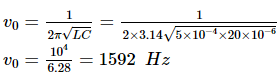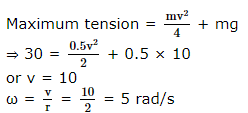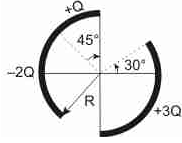Courses

# SRMJEEE Physics Mock Test - 10

## 35 Questions MCQ Test SRMJEEE Subject Wise & Full Length Mock Tests | SRMJEEE Physics Mock Test - 10

Description
This mock test of SRMJEEE Physics Mock Test - 10 for JEE helps you for every JEE entrance exam. This contains 35 Multiple Choice Questions for JEE SRMJEEE Physics Mock Test - 10 (mcq) to study with solutions a complete question bank. The solved questions answers in this SRMJEEE Physics Mock Test - 10 quiz give you a good mix of easy questions and tough questions. JEE students definitely take this SRMJEEE Physics Mock Test - 10 exercise for a better result in the exam. You can find other SRMJEEE Physics Mock Test - 10 extra questions, long questions & short questions for JEE on EduRev as well by searching above.
QUESTION: 1

Solution:
QUESTION: 2

Solution:
QUESTION: 3

### An oscillator circuit consists of an inductance of 0.5 mH and a capacitor of 20 μF. The resonance frequency of the circuit is

Solution:QUESTION: 4

An explosion blows a rock into three parts. Two parts go off at right angles to each other. These two are, 1 kg first part moving with a velocity of 12 ms−1 and 2 kg second part moving with a velocity of 8 ms−1. If the third part flies off with a velocity of 4 ms−1, its mass would be:

Solution:
QUESTION: 5

A weightless thread can support tension upto 30 N. A stone of mass 0.5 kg is tied to it and is resolved in a circular path of radius 2 m in a vertical plane. if g = 10 m/s2, then the maximum angular velocity of the stone will be

Solution:QUESTION: 6

Calculate the work done, if a wire is loaded by 'Mg' weight and the increase in length is 'l'

Solution:
QUESTION: 7

The colour of the ⁺ve column in a gas discharge tube depends upon

Solution:
QUESTION: 8

The length of a wire of a potentiometer is 100 cm, and the e.m.f. of its standard cell is E volt. It is employed to measure the e.m.f. of a battery, whose internal resistance is 0.5 Ω. If the balance point is obtained at l = 30 cm from the positive end, the e.m.f. of the battery is

Solution:
QUESTION: 9

Consider the following statement for a bridge type rectifier
1. Centre tap is not required for the secondary of the transformer.
2. The output will be half wave rectification if one of the diodes of the bridge circuit gets open circuited.
3. The bridge requires four diodes
4. If one of the diodes gets short circuited, the transformer will be short circuited.
Which of the above statements are correct?

Solution:
QUESTION: 10

The coefficient of friction between a body and the surface of an inclined plane at 45° is 0.5. If g = 9.8 m/s2, the acceleration of the body downwards in m/s2 is

Solution:
QUESTION: 11

Figure show three circular arcs, each of radius R and total charge as indicated. The net electric potential at the centre of curvature is:Solution:
QUESTION: 12

Given radius of Earth 'R' and length of a day 'T' the height of a geostationary satellite is [G - Gravitational Constant, M - Mass of Earth]

Solution:
QUESTION: 13

A thermos flask is polished well

Solution:
QUESTION: 14

If an oxygen molecule and a hydrogen molecule is having same temperature, then the ratio of their average kinetic energies will be

Solution:
QUESTION: 15

When two surfaces are coated with a lubricant, then they

Solution:
QUESTION: 16

A proton is moving in a uniform magnetic field B in a circular path of radius a in a direction perpendicular to z-axis along which field B exists. Calculate the angular momentum, if the radius is a charge on proton is e.

Solution:
QUESTION: 17

The domain formation is a necessary feature of

Solution:
QUESTION: 18

If an air-bubble of radius 0.4 mm at a depth h in water having surface tension of 72 x 10⁻3 N-m⁻1 and density 1000 kg-m⁻3 is in equilibrium then, the value of h is (Take g = 10 m-s⁻2)

Solution:
QUESTION: 19

A body projected vertically upwards with a velocity u returns to the starting point in 4 s. If g = 10 m−s−2, the value of u is

Solution:
QUESTION: 20

A body is thrown with a velocity of 9.8 m/s making an angle of 30° with the horizontal. It will hit the ground after a time

Solution:
QUESTION: 21

A child swinging on a swing in a sitting position, stands up, then the time period of the swing will

Solution:
QUESTION: 22

A force of 50 dynes is acted on a body of mass 5 g which is at an interval of 3 seconds, then impulse is

Solution:
QUESTION: 23

Two projectiles are fired at different angles with the same magnitude of velocity, such that they have the same range. At what angles they might have been projected?

Solution:
QUESTION: 24

The resolving power of a telescope depends on

Solution:
QUESTION: 25

A disc is of mass M and radius r. The moment of inertia of it about an axis tangential to its edge and in plane of the disc or parallel to its diameter is

Solution:
QUESTION: 26

The dimensional formula for torque is

Solution:
QUESTION: 27

A liquid wets a solid completely. The meniscus of the liquid in a sufficiently long tube is

Solution:
QUESTION: 28

In an elastic one dimensional collision between two particles, the relative velocity of approach before collision is:

Solution:
QUESTION: 29

e = αt-1/2 βt2, If temperature of cold junction is 0°C then temperature of inversion is (if α = 500.0μV/°C,β = 5.0μV/Square°C)

Solution:
QUESTION: 30

Two identical vessels A and B with frictionless pistons contain the same ideal gas at the same temperature and the same volume V. The masses of gas in A and B are mA and mB respectively. The gases are allowed to expand isothermally to the same final volume 3V. The change in pressure of the gas in A and B are found to be Δ P and 1.5 Δ P respectively. Then

Solution:
QUESTION: 31

A perfect gas contained in a cylinder is kept in vacuum. If the cylinder suddenly bursts, then the temperature of the gas

Solution:
QUESTION: 32

Which of the following pair has same dimensions?

Solution:
QUESTION: 33

When a source of light is receding away from an observer, then the spectral lines will get displaced towards

Solution:
QUESTION: 34

The path difference between the two waves y₁ = a₁ sin (ωt - 2πx/λ) and y₂ = a₂ cos (ωt - 2πx/λ + φ) is

Solution:
QUESTION: 35

A spring of force constant 800 N/m has an extension of 5 cm. The work done in extending it from 5 cm to 15 cm is

Solution: